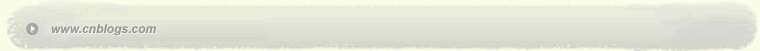Impossible is nothing 爱过知情重醉过知酒浓 　　花开花谢终是空 　　缘份不停留像春风来又走 　　女人如花花似梦

 < 2022年9月 >
28293031123
45678910
11121314151617
18192021222324
2526272829301
2345678

• 随笔 - 8
• 文章 - 91
• 评论 - 16
• 引用 - 0

# 相册

•

### 评论排行榜

##### 上次提到过为容器生成数据的问题，我给出的用 boost.lambda 的方法是 上次提到过为容器生成数据的问题，我给出的用 boost.lambda 的方法是：  std::vector<int> vect(10);  int i = 0;  std::for_each( vect.begin(), vect.end(), _1 = ++var(i) );不错，这样可以生成连续的数字，也还算比较简洁，因为代码量不会随着容器的大小而变化，不过，如果要在容器内填入随机数呢？其实比上面更简单，因为 STL 的 generate 算法就是设计来做这个的：  std::vector<int> vect(10);  std::generate(vect.begin(), vect.end(), rand);rand 是我们熟悉的标准 C 库函数，这样我们可以生成任意数量的随机数了，不过还是有点不好的地方：每次生成的序列都是一样的，因为 rand 生成的是伪随机数。这个容易解决，我们必须先 seed 一下：  std::vector<int> vect(10);  srand(time(NULL));  std::generate(vect.begin(), vect.end(), rand);好了，我们终于还是用了三行（其实是两行，声明 vector 总是必需的吧！），但是好歹是有了一个可用的方案。回头看看，前面的连续整数问题也可以用 generate 来做，方法不言而喻：  std::vector<int> vect(10);  int i = 0;  std::generate(vect.begin(), vect.end(), ++var(i));好处是 generate 本身更能说明这句话的用途，当然这个可能因人而异。我知道有人一定在问：一定要两行么？一定要有一个初始变量么？答案是可以没有，但是要用到另外的算法，再加上 boost.lambda 的协助。看看下面：  std::vector<int> vect(10);  std::partial_sum(vect.begin(), vect.end(), vect.begin(), _2 = _1 + 1);如果你现在把 vect 输出，你会得到：0 1 2 3 4 5 6 7 8 9乍看起来不太好理解，我来慢慢解释。partial_sum 的第4个参数是一个双参数的 functor ，在这里，lambda 表达式 _2 = _1 + 1 充当了这个角色，它相当于 f(x, y)  {  y  =  x  +  1;  } 而 partial_sum 呢？它把一个序列的 partial sum 送到结果序列中去，例如如果输入一个数组 v ，而输出是 r ，那么它的计算就是 r = v             r = f( r, r ) r = f( r, r ) ...... r = f( r, r ) 而当我们把 partial_sum 作用于 vect 本身，结果就成了 vect = vect                            // vect = 0 vect = (vect = vect + 1)   // vect = 1 vect = (vect = vect + 1)   // vect = 2 ...... vect = (vect = vect + 1)   // vect = 9 你一定发现其中的问题所在了：首先，我们必须依赖于编译器把 vect 初始化为0，其次，vect = vect 是不可回避的。以我当前所想到的，也只能这样了。推广一下，如果把 _2 = _1 + 1 中的常数 1 换成另外的数字，我们就可以用一句话得到从 0 开始的等差数列，例如  std::partial_sum(vect.begin(), vect.end(), vect.begin(), _2 = _1 + 3);得到的是0 3 6 9 12 15 18 21 24 27如果再发挥一点想象力，你就可以构造出更复杂的 lambda 表达式，从而得到更复杂的数组（也许这里叫数列更好吧），例如  std::partial_sum(vect.begin(), vect.end(), vect.begin(), _2 = 2 * _1 + 1);得到的是 2 的 n 次方 - 1 数列0 1 3 7 15 31 63 127 255 511在 STL 算法中，adjacent_difference 和 partial_sum 是逆运算，因此，上面的事情也可以用 adjacent_difference 来做，只不过要把 lambda 表达式中的参数位置换一下，例如要得到 0, 3, 6... 的等差数列，只需要  std::adjacent_difference(vect.begin(), vect.end(), vect.begin(), _1 = _2 + 3);而 2 的 n 次方 - 1 数列也是同样道理  std::adjacent_difference(vect.begin(), vect.end(), vect.begin(), _1 = 2*_2 + 1);如果你要生成倒序的数列呢？当然，STL 算法 reverse 可以派上用场，不过也不要忘了 STL 还有 reverse_iterator 这回事，用它就无需另外调用 reverse 了：  std::partial_sum(vect.rbegin(), vect.rend(), vect.rbegin(), _2 = 2*_1 + 1);得到511 255 127 63 31 15 7 3 1 0最后还要提醒大家不要忘了一个很有用的 STL 算法： random_shuffle 。它可以把 Random access container 里面的值打乱，配合上面的数列生成，在很多场合是进行测试 （例如测试排序算法） 的好工具。在我的机器上，下面两行  std::partial_sum(vect.begin(), vect.end(), vect.begin(), _2 = 2*_1 + 1);  std::random_shuffle(vect.begin(), vect.end());得到打乱以后的数列：255 1 511 3 0 31 127 7 15 63 =================================================================================有了强大的生成机制作基础，下面的实验也更加容易了。STL 的 count_if 和 find_if 都接受一个 predicate 作为比较的依据，而这个 predicate 往往非常简单，以至于为它专门写一个 functor 简直不可接受。在第一篇里面已经展示了用 boost.lambda 生成临时的无名 functor 的能力，这里再多说一点。下面先生成 2^n - 1 的数组，然后找出其中第一个大于100的数  std::vector<int> vect(10);  std::partial_sum(vect.begin(), vect.end(), vect.begin(), _2 = 2*_1 + 1);    std::cout << *std::find_if(vect.begin(), vect.end(), _1 > 100);输出为 127 ，如我们所料。同样道理，如果是 count_if ，则会得到大于100的数的个数  std::cout << std::count_if(vect.begin(), vect.end(), _1 > 100);输出是 3 。注意细节：find_if 返回一个 iterator ，所以在它之前有 * 解引用，而 count_if 直接返回一个数字，无需解引用。与之类似的还有 STL 的 partition 算法，它根据传入的 predicate 对一个序列进行划分，predicate 得到 true 的将放在前面，其余的放在后面，返回的是那些“ 放在 后面”的元素中的第一个，换言之就是分界点。下面的代码  std::vector<int> vect(10);  std::partial_sum(vect.begin(), vect.end(), vect.begin(), _2 = 2*_1 + 1);    std::cout << *std::partition(vect.begin(), vect.end(), _1 > 100) << std::endl;    std::for_each(vect.begin(), vect.end(), std::cout << _1 << " ");输出为7511 255 127 7 15 31 63 3 1 0如果仔细观察，还可以发现上面的输出有点问题：数列中原有的顺序（0, 1, 3, 7...）不复存在，这是因为 partition 并不是一个稳定排序的算法，它不保证排序结果保有原来的顺序。如果需要稳定排序，可以使用 stable_partition 。只需要更改排序的那一句代码为  std::cout << *std::stable_partition(vect.begin(), vect.end(), _1 > 100) << std::endl;结果是0127 255 511 0 1 3 7 15 31 63当然，如果你还记得大学里的算法理论，就知道它们在效率上是有点区别的，partition 的复杂度保证为 O(n) ，具体地说是保证不超过 n/2 次交换；而 stable_partition 在最好情况下为 O(n) ，最差情况则达到 O(n*log(n)) 。顺便说一下，上面的几件简单的事情，用标准的 STL 算法都可以办到，只不过实在是……面目可憎：  std::cout << *std::partition(vect.begin(), vect.end(),     std::bind2nd(std::greater<int>(), 100)) << std::endl;这句代码做的事情和前面的 partition 一模一样，但是孰优孰劣，大家自有公断。
posted on 2006-06-28 13:44 笑笑生 阅读(139) 评论(0)  编辑 收藏 引用Copyright © 笑笑生 Powered by: 博客园 模板提供：沪江博客